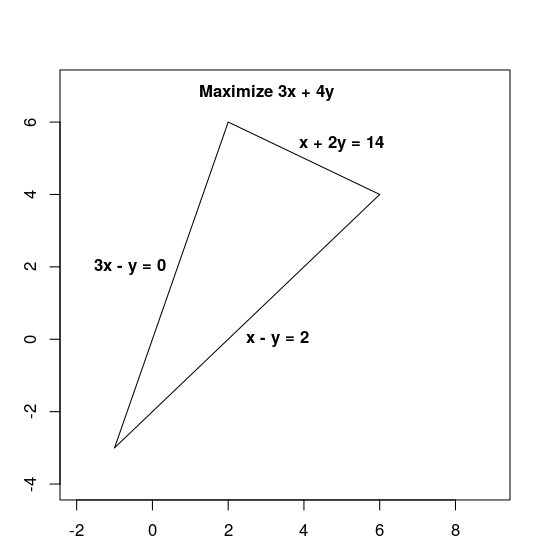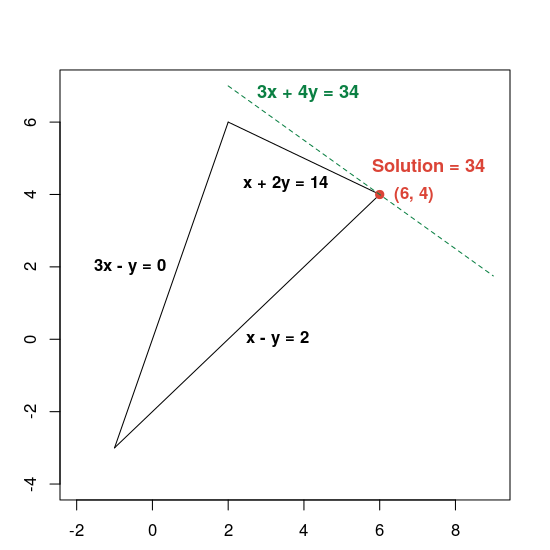# Solving an LP Problem

The following sections present an example of an LP problem and show how to solve it. Here's the problem:

Maximize `3x + 4y` subject to the following constraints:

1. `x + 2y` ≤ 14
2. `3x - y` ≥ 0
3. `x - y` ≤ 2

Both the objective function, `3x + 4y`, and the constraints are given by linear expressions, which makes this a linear problem.

The constraints define the feasible region, which is the triangle shown below, including its interior.## Basic steps for solving an LP problem

To solve a LP problem, your program should include the following steps:

1. Import the linear solver wrapper,
2. declare the LP solver,
3. define the variables,
4. define the constraints,
5. define the objective,
6. call the LP solver; and
7. display the solution

## Solution using the MPSolver

The following section present a program that solves the problem using the MPSolver wrapper and an LP solver.

Note. To run the program below, you need to install OR-Tools.

The primary OR-Tools linear optimization solver is Glop, Google's in-house linear programming solver. It's fast, memory efficient, and numerically stable.

### Import the linear solver wrapper

Import (or include) the OR-Tools linear solver wrapper, an interface for MIP solvers and linear solvers, as shown below.

### Python

`from ortools.linear_solver import pywraplp`

### C++

```#include <iostream>
#include <memory>

#include "ortools/linear_solver/linear_solver.h"```

### Java

```import com.google.ortools.Loader;

### C#

```using System;

### Declare the LP solver

`MPsolver` is a wrapper for several different solvers, including Glop. The code below declares the GLOP solver.

### Python

```solver = pywraplp.Solver.CreateSolver("GLOP")
if not solver:
return```

### C++

```std::unique_ptr<MPSolver> solver(MPSolver::CreateSolver("SCIP"));
if (!solver) {
LOG(WARNING) << "SCIP solver unavailable.";
return;
}```

### Java

`MPSolver solver = MPSolver.createSolver("GLOP");`

### C#

```Solver solver = Solver.CreateSolver("GLOP");
if (solver is null)
{
return;
}```

Note: Substitute `PDLP` for `GLOP` to use an alternative LP solver. For more details on choosing solvers, see advanced LP solving, and for installation of third-party solvers, see the installation guide.

### Create the variables

First, create variables x and y whose values are in the range from 0 to infinity.

### Python

```x = solver.NumVar(0, solver.infinity(), "x")
y = solver.NumVar(0, solver.infinity(), "y")

print("Number of variables =", solver.NumVariables())```

### C++

```const double infinity = solver->infinity();
// x and y are non-negative variables.
MPVariable* const x = solver->MakeNumVar(0.0, infinity, "x");
MPVariable* const y = solver->MakeNumVar(0.0, infinity, "y");
LOG(INFO) << "Number of variables = " << solver->NumVariables();```

### Java

```double infinity = java.lang.Double.POSITIVE_INFINITY;
// x and y are continuous non-negative variables.
MPVariable x = solver.makeNumVar(0.0, infinity, "x");
MPVariable y = solver.makeNumVar(0.0, infinity, "y");
System.out.println("Number of variables = " + solver.numVariables());```

### C#

```Variable x = solver.MakeNumVar(0.0, double.PositiveInfinity, "x");
Variable y = solver.MakeNumVar(0.0, double.PositiveInfinity, "y");

Console.WriteLine("Number of variables = " + solver.NumVariables());```

### Define the constraints

Next, define the constraints on the variables. Give each constraint a unique name (such as `constraint0`), and then define the coefficients for the constraint.

### Python

```# Constraint 0: x + 2y <= 14.
solver.Add(x + 2 * y <= 14.0)

# Constraint 1: 3x - y >= 0.
solver.Add(3 * x - y >= 0.0)

# Constraint 2: x - y <= 2.

print("Number of constraints =", solver.NumConstraints())```

### C++

```// x + 2*y <= 14.
MPConstraint* const c0 = solver->MakeRowConstraint(-infinity, 14.0);
c0->SetCoefficient(x, 1);
c0->SetCoefficient(y, 2);

// 3*x - y >= 0.
MPConstraint* const c1 = solver->MakeRowConstraint(0.0, infinity);
c1->SetCoefficient(x, 3);
c1->SetCoefficient(y, -1);

// x - y <= 2.
MPConstraint* const c2 = solver->MakeRowConstraint(-infinity, 2.0);
c2->SetCoefficient(x, 1);
c2->SetCoefficient(y, -1);
LOG(INFO) << "Number of constraints = " << solver->NumConstraints();```

### Java

```// x + 2*y <= 14.
MPConstraint c0 = solver.makeConstraint(-infinity, 14.0, "c0");
c0.setCoefficient(x, 1);
c0.setCoefficient(y, 2);

// 3*x - y >= 0.
MPConstraint c1 = solver.makeConstraint(0.0, infinity, "c1");
c1.setCoefficient(x, 3);
c1.setCoefficient(y, -1);

// x - y <= 2.
MPConstraint c2 = solver.makeConstraint(-infinity, 2.0, "c2");
c2.setCoefficient(x, 1);
c2.setCoefficient(y, -1);
System.out.println("Number of constraints = " + solver.numConstraints());```

### C#

```// x + 2y <= 14.
solver.Add(x + 2 * y <= 14.0);

// 3x - y >= 0.
solver.Add(3 * x - y >= 0.0);

// x - y <= 2.

Console.WriteLine("Number of constraints = " + solver.NumConstraints());```

### Define the objective function

The following code defines the objective function, `3x + 4y`, and specifies that this is a maximization problem.

### Python

```# Objective function: 3x + 4y.
solver.Maximize(3 * x + 4 * y)```

### C++

```// Objective function: 3x + 4y.
MPObjective* const objective = solver->MutableObjective();
objective->SetCoefficient(x, 3);
objective->SetCoefficient(y, 4);
objective->SetMaximization();```

### Java

```// Maximize 3 * x + 4 * y.
MPObjective objective = solver.objective();
objective.setCoefficient(x, 3);
objective.setCoefficient(y, 4);
objective.setMaximization();```

### C#

```// Objective function: 3x + 4y.
solver.Maximize(3 * x + 4 * y);```

### Invoke the solver

The following code invokes the solver.

### Python

`status = solver.Solve()`

### C++

```const MPSolver::ResultStatus result_status = solver->Solve();
// Check that the problem has an optimal solution.
if (result_status != MPSolver::OPTIMAL) {
LOG(FATAL) << "The problem does not have an optimal solution!";
}```

### Java

`final MPSolver.ResultStatus resultStatus = solver.solve();`

### C#

`Solver.ResultStatus resultStatus = solver.Solve();`

### Display the solution

The following code displays the solution.

### Python

```if status == pywraplp.Solver.OPTIMAL:
print("Solution:")
print("Objective value =", solver.Objective().Value())
print("x =", x.solution_value())
print("y =", y.solution_value())
else:
print("The problem does not have an optimal solution.")```

### C++

```LOG(INFO) << "Solution:";
LOG(INFO) << "Optimal objective value = " << objective->Value();
LOG(INFO) << x->name() << " = " << x->solution_value();
LOG(INFO) << y->name() << " = " << y->solution_value();```

### Java

```if (resultStatus == MPSolver.ResultStatus.OPTIMAL) {
System.out.println("Solution:");
System.out.println("Objective value = " + objective.value());
System.out.println("x = " + x.solutionValue());
System.out.println("y = " + y.solutionValue());
} else {
System.err.println("The problem does not have an optimal solution!");
}```

### C#

```// Check that the problem has an optimal solution.
if (resultStatus != Solver.ResultStatus.OPTIMAL)
{
Console.WriteLine("The problem does not have an optimal solution!");
return;
}
Console.WriteLine("Solution:");
Console.WriteLine("Objective value = " + solver.Objective().Value());
Console.WriteLine("x = " + x.SolutionValue());
Console.WriteLine("y = " + y.SolutionValue());```

### The complete programs

The complete programs are shown below.

### Python

```from ortools.linear_solver import pywraplp

def LinearProgrammingExample():
"""Linear programming sample."""
# Instantiate a Glop solver, naming it LinearExample.
solver = pywraplp.Solver.CreateSolver("GLOP")
if not solver:
return

# Create the two variables and let them take on any non-negative value.
x = solver.NumVar(0, solver.infinity(), "x")
y = solver.NumVar(0, solver.infinity(), "y")

print("Number of variables =", solver.NumVariables())

# Constraint 0: x + 2y <= 14.
solver.Add(x + 2 * y <= 14.0)

# Constraint 1: 3x - y >= 0.
solver.Add(3 * x - y >= 0.0)

# Constraint 2: x - y <= 2.

print("Number of constraints =", solver.NumConstraints())

# Objective function: 3x + 4y.
solver.Maximize(3 * x + 4 * y)

# Solve the system.
status = solver.Solve()

if status == pywraplp.Solver.OPTIMAL:
print("Solution:")
print("Objective value =", solver.Objective().Value())
print("x =", x.solution_value())
print("y =", y.solution_value())
else:
print("The problem does not have an optimal solution.")

print("Problem solved in %f milliseconds" % solver.wall_time())
print("Problem solved in %d iterations" % solver.iterations())

LinearProgrammingExample()```

### C++

```#include <iostream>
#include <memory>

#include "ortools/linear_solver/linear_solver.h"

namespace operations_research {
void LinearProgrammingExample() {
std::unique_ptr<MPSolver> solver(MPSolver::CreateSolver("SCIP"));
if (!solver) {
LOG(WARNING) << "SCIP solver unavailable.";
return;
}

const double infinity = solver->infinity();
// x and y are non-negative variables.
MPVariable* const x = solver->MakeNumVar(0.0, infinity, "x");
MPVariable* const y = solver->MakeNumVar(0.0, infinity, "y");
LOG(INFO) << "Number of variables = " << solver->NumVariables();

// x + 2*y <= 14.
MPConstraint* const c0 = solver->MakeRowConstraint(-infinity, 14.0);
c0->SetCoefficient(x, 1);
c0->SetCoefficient(y, 2);

// 3*x - y >= 0.
MPConstraint* const c1 = solver->MakeRowConstraint(0.0, infinity);
c1->SetCoefficient(x, 3);
c1->SetCoefficient(y, -1);

// x - y <= 2.
MPConstraint* const c2 = solver->MakeRowConstraint(-infinity, 2.0);
c2->SetCoefficient(x, 1);
c2->SetCoefficient(y, -1);
LOG(INFO) << "Number of constraints = " << solver->NumConstraints();

// Objective function: 3x + 4y.
MPObjective* const objective = solver->MutableObjective();
objective->SetCoefficient(x, 3);
objective->SetCoefficient(y, 4);
objective->SetMaximization();

const MPSolver::ResultStatus result_status = solver->Solve();
// Check that the problem has an optimal solution.
if (result_status != MPSolver::OPTIMAL) {
LOG(FATAL) << "The problem does not have an optimal solution!";
}

LOG(INFO) << "Solution:";
LOG(INFO) << "Optimal objective value = " << objective->Value();
LOG(INFO) << x->name() << " = " << x->solution_value();
LOG(INFO) << y->name() << " = " << y->solution_value();
}
}  // namespace operations_research

int main(int argc, char** argv) {
operations_research::LinearProgrammingExample();
return EXIT_SUCCESS;
}```

### Java

```package com.google.ortools.linearsolver.samples;

/** Simple linear programming example. */
public final class LinearProgrammingExample {
public static void main(String[] args) {
MPSolver solver = MPSolver.createSolver("GLOP");

double infinity = java.lang.Double.POSITIVE_INFINITY;
// x and y are continuous non-negative variables.
MPVariable x = solver.makeNumVar(0.0, infinity, "x");
MPVariable y = solver.makeNumVar(0.0, infinity, "y");
System.out.println("Number of variables = " + solver.numVariables());

// x + 2*y <= 14.
MPConstraint c0 = solver.makeConstraint(-infinity, 14.0, "c0");
c0.setCoefficient(x, 1);
c0.setCoefficient(y, 2);

// 3*x - y >= 0.
MPConstraint c1 = solver.makeConstraint(0.0, infinity, "c1");
c1.setCoefficient(x, 3);
c1.setCoefficient(y, -1);

// x - y <= 2.
MPConstraint c2 = solver.makeConstraint(-infinity, 2.0, "c2");
c2.setCoefficient(x, 1);
c2.setCoefficient(y, -1);
System.out.println("Number of constraints = " + solver.numConstraints());

// Maximize 3 * x + 4 * y.
MPObjective objective = solver.objective();
objective.setCoefficient(x, 3);
objective.setCoefficient(y, 4);
objective.setMaximization();

final MPSolver.ResultStatus resultStatus = solver.solve();

if (resultStatus == MPSolver.ResultStatus.OPTIMAL) {
System.out.println("Solution:");
System.out.println("Objective value = " + objective.value());
System.out.println("x = " + x.solutionValue());
System.out.println("y = " + y.solutionValue());
} else {
System.err.println("The problem does not have an optimal solution!");
}

System.out.println("Problem solved in " + solver.wallTime() + " milliseconds");
System.out.println("Problem solved in " + solver.iterations() + " iterations");
}

private LinearProgrammingExample() {}
}```

### C#

```using System;

public class LinearProgrammingExample
{
static void Main()
{
Solver solver = Solver.CreateSolver("GLOP");
if (solver is null)
{
return;
}
// x and y are continuous non-negative variables.
Variable x = solver.MakeNumVar(0.0, double.PositiveInfinity, "x");
Variable y = solver.MakeNumVar(0.0, double.PositiveInfinity, "y");

Console.WriteLine("Number of variables = " + solver.NumVariables());

// x + 2y <= 14.
solver.Add(x + 2 * y <= 14.0);

// 3x - y >= 0.
solver.Add(3 * x - y >= 0.0);

// x - y <= 2.

Console.WriteLine("Number of constraints = " + solver.NumConstraints());

// Objective function: 3x + 4y.
solver.Maximize(3 * x + 4 * y);

Solver.ResultStatus resultStatus = solver.Solve();

// Check that the problem has an optimal solution.
if (resultStatus != Solver.ResultStatus.OPTIMAL)
{
Console.WriteLine("The problem does not have an optimal solution!");
return;
}
Console.WriteLine("Solution:");
Console.WriteLine("Objective value = " + solver.Objective().Value());
Console.WriteLine("x = " + x.SolutionValue());
Console.WriteLine("y = " + y.SolutionValue());

Console.WriteLine("Problem solved in " + solver.WallTime() + " milliseconds");
Console.WriteLine("Problem solved in " + solver.Iterations() + " iterations");
}
}```

### Optimal solution

The program returns the optimal solution to the problem, as shown below.

``````Number of variables = 2
Number of constraints = 3
Solution:
x = 6.0
y = 4.0
Optimal objective value = 34.0
``````

Here is a graph showing the solution:The dashed green line is defined by setting the objective function equal to its optimal value of 34. Any line whose equation has the form `3x + 4y = c` is parallel to the dashed line, and 34 is the largest value of c for which the line intersects the feasible region.